## Strip or remove non-numeric characters from text strings

Sometimes, you may need to remove all of the non-numeric characters from the text strings, and only keep the numbers as below screenshot shown. This article will introduce some formulas for solving this task in Excel.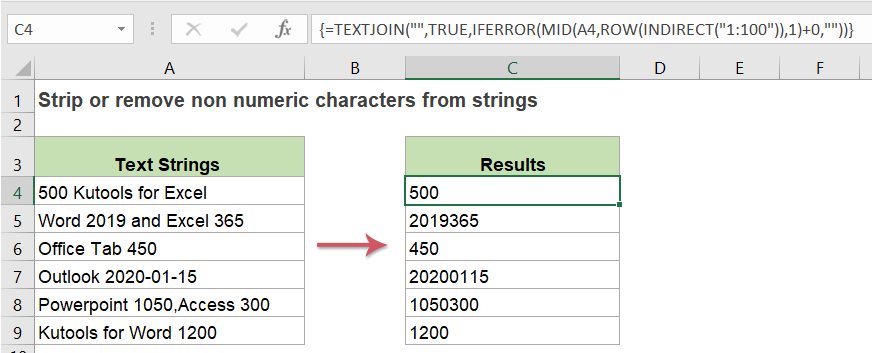#### Strip or remove all non-numeric characters from text strings with formulas

In Excel 2019 and Office 365, a new TEXTJOIN function combining with the IFERROR, MID, ROW and INDIRECT functions can help you to extract only numbers from a text string, the generic syntax is:

=TEXTJOIN("",TRUE,IFERROR(MID(text,ROW(INDIRECT("1:100")),1)+0,""))
• text:the text string or cell value that you want to remove all non-numeric characters from.

1. Please copy or enter the below formula into a blank cell where you want to output the result:

=TEXTJOIN("",TRUE,IFERROR(MID(A2,ROW(INDIRECT("1:100")),1)+0,""))

2. And then, press Ctrl + Shift + Enter keys together to get the first result, see screenshot: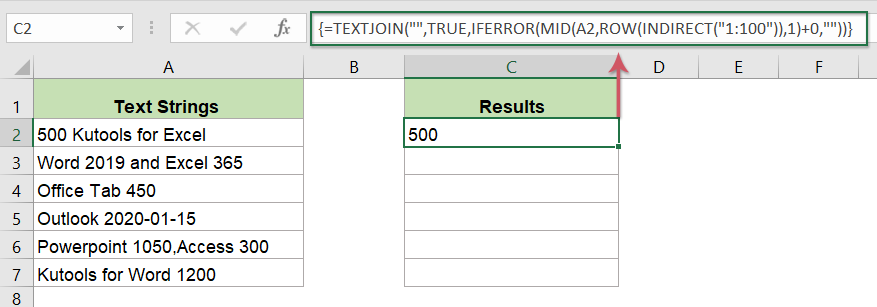3. Then, select the formula cell, and then drag the fill handle down to the cells that you want to apply this formula, only numbers have been extracted, and all other non-numeric characters have been stripped, see screenshot: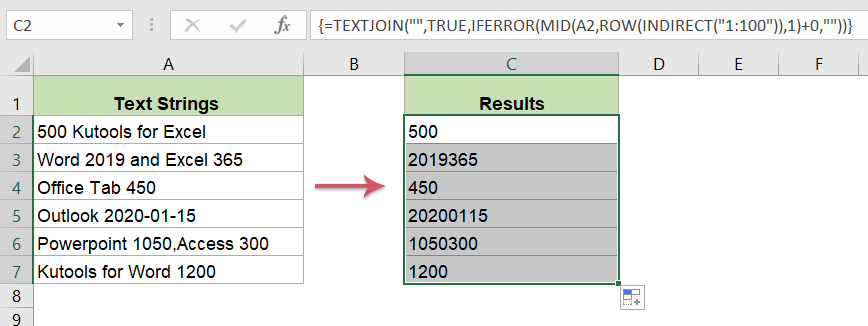##### Explanation of the formula:

ROW(INDIRECT("1:100")：Number 1:100 in the INDIRECT formula means the MID function evaluates 100 characters of the text string. This array will contain 100 numbers like this: {1;2;3;4;5;6;7;8....98;99;100}.
Note: If your text string is much longer, you can change the number 100 to larger number as you need.

MID(A2,ROW(INDIRECT("1:100")),1: This MID function is used to extract text in cell A2 to get one character, and it will an array like this:
{"5";"0";"0";" ";"K";"u";"t";"o";"o";"l";"s";" ";"f";"o";"r";" ";"E";"x";"c";"e";"l";"";"";"";"";"";""...}

MID(A2,ROW(INDIRECT("1:100")),1)+0: Adding 0 value after this array is used to force the text to a number, the numeric text value will be converted to number, and non-numeric values will be displayed as #VALUE error value like this:
{"5";"0";"0";#VALUE!;#VALUE!;#VALUE!;#VALUE!;#VALUE!;#VALUE! !;#VALUE! !;#VALUE!...}

IFERROR(MID(A2,ROW(INDIRECT("1:100")),1)+0: This IFERROR function is used to replace all of the error values with an empty string like this:
{"5"; "0";"0";"";""; "";"";"";"";"";""; … }

TEXTJOIN("",TRUE,IFERROR(MID(A2,ROW(INDIRECT("1:100")),1)+0,"")): At last, this TEXTJION function will combine all non-empty values in the array which returned by the IFFERROR function and returns the result.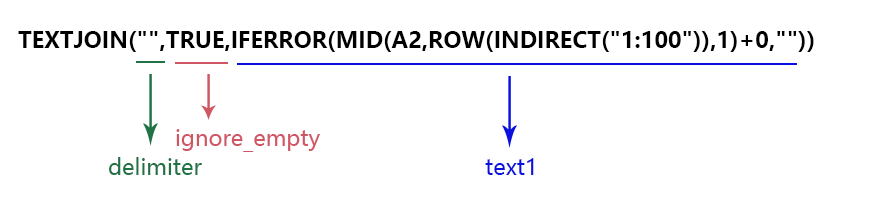##### Notes:

1. With the above formula, the numbers will be returned as text format, if you need a real numeric value, please apply this formula, remember to press Ctrl + Shift + Enter keys together to get the correct result.

=TEXTJOIN("",TRUE,IFERROR(MID(A2,ROW(INDIRECT("1:100")),1)+0,""))+0

2. In early versions Excel, this formula will not work, in this case, the following formula may help you, please copy or enter this formula into a blank cell:

=SUMPRODUCT(MID(0&A2, LARGE(INDEX(ISNUMBER(--MID(A2, ROW(INDIRECT("1:"&LEN(A2))), 1)) * ROW(INDIRECT("1:"&LEN(A2))), 0), ROW(INDIRECT("1:"&LEN(A2))))+1, 1) * 10^ROW(INDIRECT("1:"&LEN(A2)))/10)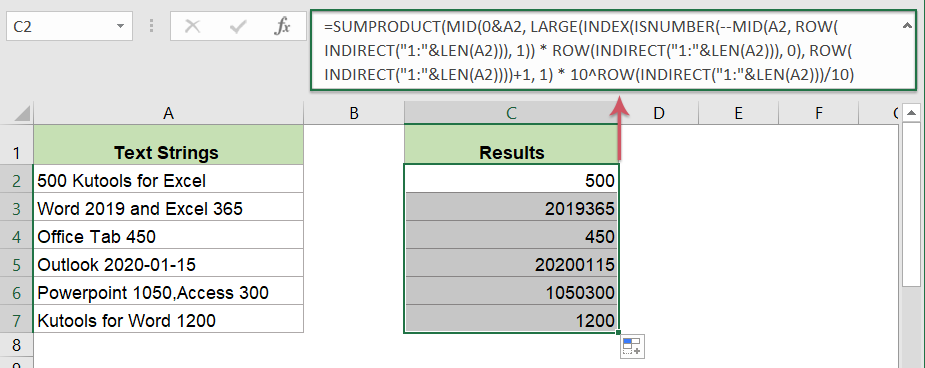#### Strip or remove all non-numeric characters from text strings with an easy feature

Maybe, it is too long to remember the above formulas, here, I will introduce the Kutools for Excel for you, with its Remove Characters feature, you can remove numeric, alphabetic, non-printable or alphanumeric characters from text strings with only several clicks. Click to download Kutools for Excel for free trial!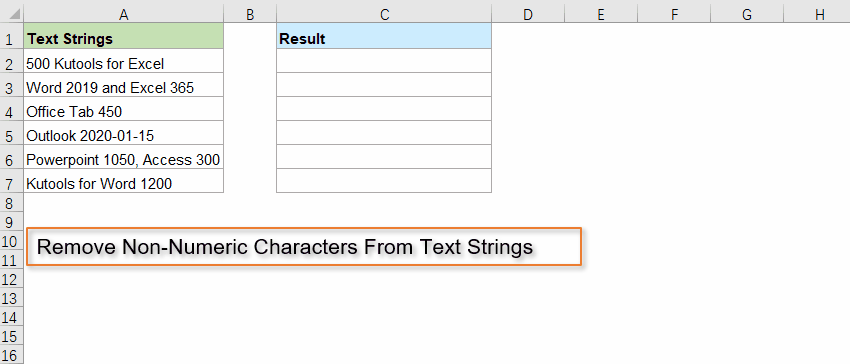#### Relative functions used:

• TEXTJOIN:
• The TEXTJOIN function joins multiple values from a row, column or a range of cells with specific delimiter.
• MID:
• The MID function is used to find and return a specific number of characters from the middle of given text string.
• ROW:
• The Excel ROW function returns the row number of a reference.
• INDIRECT:
• The Excel INDIRECT function converts a text string to a valid reference.
• IFERROR:
• The IFERROR function is used to return a custom result when a formula evaluates an error, and return a normal result when no error is occurred.

#### More articles:

• Remove Line Breaks From Cells In Excel
• This tutorial provides three formulas to help you removing line breaks (which are occurred by pressing Alt + Enter keys in a cell) from specific cells in Excel.

### The Best Office Productivity Tools

#### Kutools for Excel - Helps You To Stand Out From Crowd

 Popular Features: Find, Highlight or Identify Duplicates  |  Delete Blank Rows  |  Combine Columns or Cells without Losing Data  |  Round without Formula ... Super VLookup: Multiple Criteria  |  Multiple Value  |  Across Multi-Sheets  |  Fuzzy Lookup... Adv. Drop-down List: Easy Drop Down List  |  Dependent Drop Down List  |  Multi-select Drop Down List... Column Manager: Add a Specific Number of Columns  |  Move Columns  |  Toggle Visibility Status of Hidden Columns  |  Compare Columns to Select Same & Different Cells ... Featured Features: Grid Focus  |  Design View  |  Big Formula Bar  |  Workbook & Sheet Manager | Resource Library (Auto Text)  |  Date Picker  |  Combine Worksheets  |  Encrypt/Decrypt Cells  |  Send Emails by List  |  Super Filter  |  Special Filter (filter bold/italic/strikethrough...) ... Top 15 Toolsets:  12 Text Tools (Add Text, Remove Characters ...)  |  50+ Chart Types (Gantt Chart ...)  |  40+ Practical Formulas (Calculate age based on birthday ...)  |  19 Insertion Tools (Insert QR Code, Insert Picture from Path ...)  |  12 Conversion Tools (Numbers to Words, Currency Conversion ...)  |  7 Merge & Split Tools (Advanced Combine Rows, Split Excel Cells ...)  |  ... and more

Kutools for Excel Boasts Over 300 Features, Ensuring That What You Need is Just A Click Away...#### Office Tab - Enable Tabbed Reading and Editing in Microsoft Office (include Excel)

• One second to switch between dozens of open documents!
• Reduce hundreds of mouse clicks for you every day, say goodbye to mouse hand.
• Increases your productivity by 50% when viewing and editing multiple documents.
• Brings Efficient Tabs to Office (include Excel), Just Like Chrome, Edge and Firefox.No ratings yet. Be the first to rate!
This comment was minimized by the moderator on the site
What is this formula for french settings?
This comment was minimized by the moderator on the site
Thanks for this. Nice formula.How would I alter it so that if the cell contains only letters the formula enters a 0 the results cell (rather than just blank as it is at the moment)?Thought I might be able to do it by wrapping the formula in another IF statement but I’m not getting very far.
This comment was minimized by the moderator on the site
Hello, Glenn,To display the results as blanks rather than zeros, please apply the following formula:=IF(SUM(LEN(A2)-LEN(SUBSTITUTE(A2, {"0","1","2","3","4","5","6","7","8","9"}, "")))>0, SUMPRODUCT(MID(0&A2, LARGE(INDEX(ISNUMBER(--MID(A2,ROW(INDIRECT("\$1:\$"&LEN(A2))),1))* ROW(INDIRECT("\$1:\$"&LEN(A2))),0), ROW(INDIRECT("\$1:\$"&LEN(A2))))+1,1) * 10^ROW(INDIRECT("\$1:\$"&LEN(A2)))/10),"")